﻿ 预应力矮肋T梁桥跨中横隔梁力学性能及计算方法分析

# 预应力矮肋T梁桥跨中横隔梁力学性能及计算方法分析Analysis of Mechanical Properties and Calculation Method of Mid-Span Transverse Beam of Prestressed Low-Ribbed T-Beam Bridge

Abstract: Due to the small structural height of the prestressed low-ribbed t-beam bridge and the small torsional stiffness of the main beam, the transverse distribution of the force on the bridge will be different from that of the ordinary t-beam when the bridge is under stress, and the mechanical properties of the transverse beam will also change. In order to understand the mechanical properties of the mid-span transverse beam of the prestressed low-ribbed T beam bridge and master the reasonable calculation method of the mid-span transverse beam of the prestressed low-ribbed T beam bridge, based on the finite element method of the whole bridge and the theory of eccentric pressure, this paper analyzes the mechanical behavior of the mid-span transverse beam of the prestressed concrete low-ribbed t-beam bridge, and then makes a comparative analysis with the field test results. The results show that the cross section stress is linearly distributed along with the beam height. The maximum error of the finite element method is 9.4% and the minimum error is 1.5%. The maximum error of the eccentric pressure method is 27.7% and the minimum error is 10.5%. The neutral axis position calculated by eccentric pressure method deviates from the actual neutral axis position. It is concluded that the transverse beam is in good working condition. The finite element analysis method of the whole bridge can well simulate the mechanical behavior of the transverse beam of prestressed low-ribbed t-beam bridge. It is not suitable to calculate the internal force of the transverse beam of the prestressed t-beam bridge by using the theory of eccentric pressure. The theoretical calculation of eccentric pressure method is not accurate enough, and the reason is that the effective flange width calculation of the compression flange plate is not reasonable enough.

1. 引言

2. 试验研究

2.1. 试验加载工况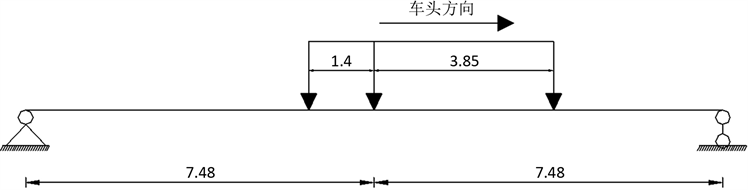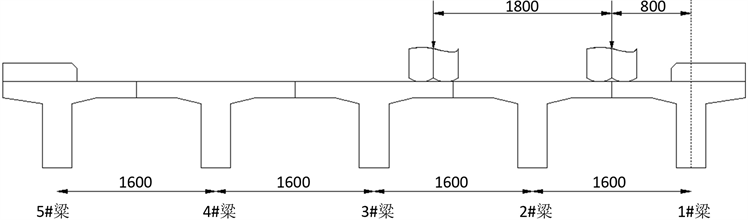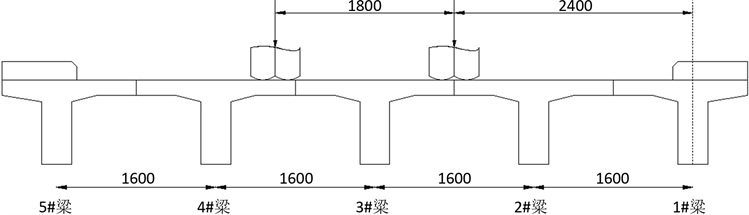2.2. 测点布置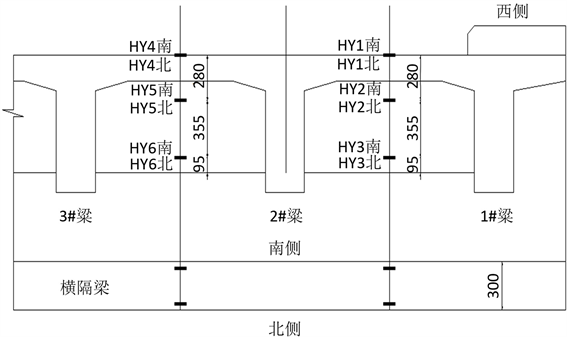Figure 4. Arrangement of measuring points

3. 理论分析方法

3.1. 全桥有限元分析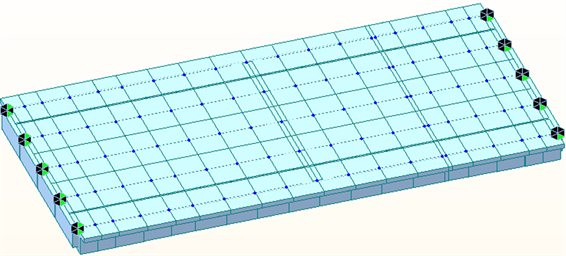Figure 5. Finite element modelTable 1. Design parameters of T beamTable 2. Geometric characteristic parameters of T beam sectionTable 3. T beam steel wire bundle

3.2. 偏心压力法

3.2.1. 荷载横向分布影响线

${\eta }_{ik}=\frac{1}{n}±\frac{{\alpha }_{i}{\alpha }_{k}}{\underset{i=1}{\overset{n}{\sum }}{\alpha }_{i}^{2}}$ (1)

${\eta }_{ik}$ ：当P = 1位于第k号主梁轴上时，对i号主梁的总作用。

${\alpha }_{i}$ ：各片主梁梁轴到截面形心的距离。

3.2.2. 横隔梁计算荷载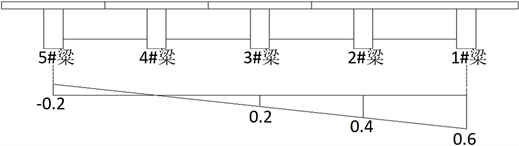Figure 6. Influence line of transverse distribution of load on 1# beam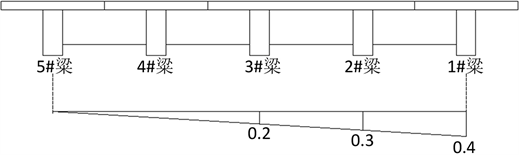Figure 7. Influence line of transverse distribution of load on 2#

3.2.3. 横隔梁计算荷载

${M}_{r}\underset{左}{\sum }{R}_{i}{b}_{i}-e$ (2)

${M}_{r}\underset{左}{\sum }{R}_{i}{b}_{i}$ (3)

$\underset{左}{\sum }{R}_{i}$ ：所求截面以左全部支撑反力 ${R}_{i}$ 的总和，支撑反力 ${R}_{i}$ 可有2.3.1节绘制的荷载横向分布影响线求得。

${b}_{i}$ ：支撑反力 ${R}_{i}$ 至所求截面距离。

e：荷载P = 1至所求截面距离。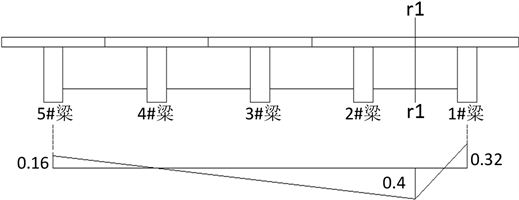Figure 8. r1 section internal force influence line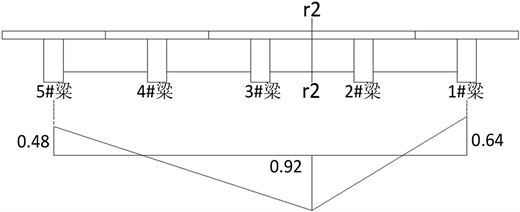Figure 9. r2 section internal force influence line

3.2.4. 横隔梁截面应力计算

$\sigma =\frac{M\cdot y}{I}$ (4)

M：截面弯矩，Mr1 = 140 KN∙m，Mr2 = 272 KN∙m。

y：截面中性轴至所求应力点的垂直距离。

I：截面惯性矩。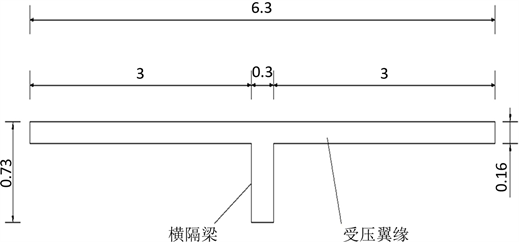Figure 10. Stress calculation cross section of transverse beam

4. 计算结果及对比分析Table 4. Stress comparison in working condition 1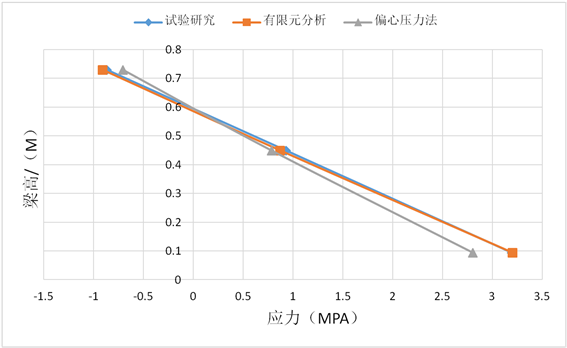Figure 11. Comparative analysis of stress in working condition 1Table 5. Stress comparison in working condition 2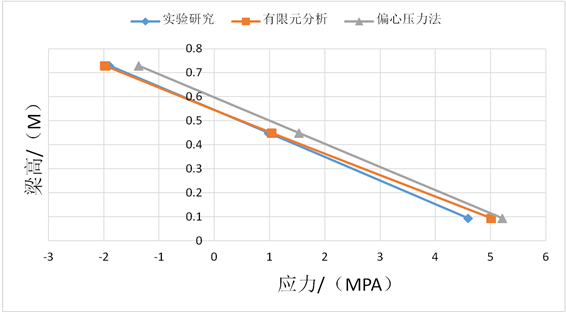Figure 12. Comparative analysis of stress in working condition 2

5. 结论

1) 横隔梁受力时应力沿梁高成线性分布，横隔梁受力均匀，工作状态良好。

2) 全桥有限元分析方法可很好的模拟预应力矮肋T梁桥横隔梁的受力性能，理论计算的横隔梁的应力与试验结果吻合，误差小于9.4%，计算精度高，可用于实际工程设计当中。

3) 采用偏心压力法理论对预应力矮肋T梁桥的横隔梁进行内力计算是不太适用的，计算精度不高，不能真实的反应梁的实际受力情况。

4) 采用偏心压力法理论计算不够精确的原因是受压翼缘板的有效翼缘宽度计算不够合理。

 黄侨, 金秀辉. 预应力混凝土矮肋斜T梁桥的计算方法研究[J]. 公路交通科技, 2005, 22(5): 79-82.

 金秀辉, 黄侨. 矮肋T形斜梁桥的组合有限元分析与试验研究[J]. 公路, 2006(10): 11-14.

 邢世玲, 徐秀丽, 李枝军, 李翊策. 墩–梁固结的曲线梁桥预应力作用试验研究[J]. 桥梁建设, 2018, 48(2): 49-54.

 席进. 低高度密肋式T梁结构参数化分析研究[J]. 桥隧工程, 2012(4): 256-260.

 刘钟仁, 刘永前, 彭鹏. 16 m低高度钢筋钢筋混凝土T梁单线铁路桥动力性能试验研究[J]. 国防交通工程与技术, 2008, 6(6): 29-30+41.

 余波, 张杰. 预应力T梁桥跨中横隔梁力学性能分析[J]. 公路, 2015, 60(7): 110-112.

 邵旭东, 顾安邦. 桥梁工程[M]. 北京: 人民交通出版社, 2016.

 中华人民共和国交通部. JTGD62-2004公路钢筋混凝土及预应力混凝土桥涵设计规范[S]. 北京: 人民交通出版社, 2004.

Top